# added to 51.80 mL of water. Calculate A solution is prepared by dissolving 22.99 of ammonium...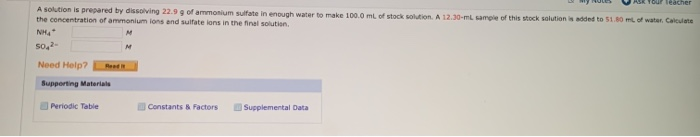added to 51.80 mL of water. Calculate A solution is prepared by dissolving 22.99 of ammonium sulfate in enough water to make 100.0 ml of stock solution. A 12.30-ml sample of this Mock solution the concentration of ammonium ions and sulfate lons in the final solution NM SO, Need Help? Red Supporting Materiale Periodic Table Constants & Factors Supplemental Data

The above problem can be solved as follows :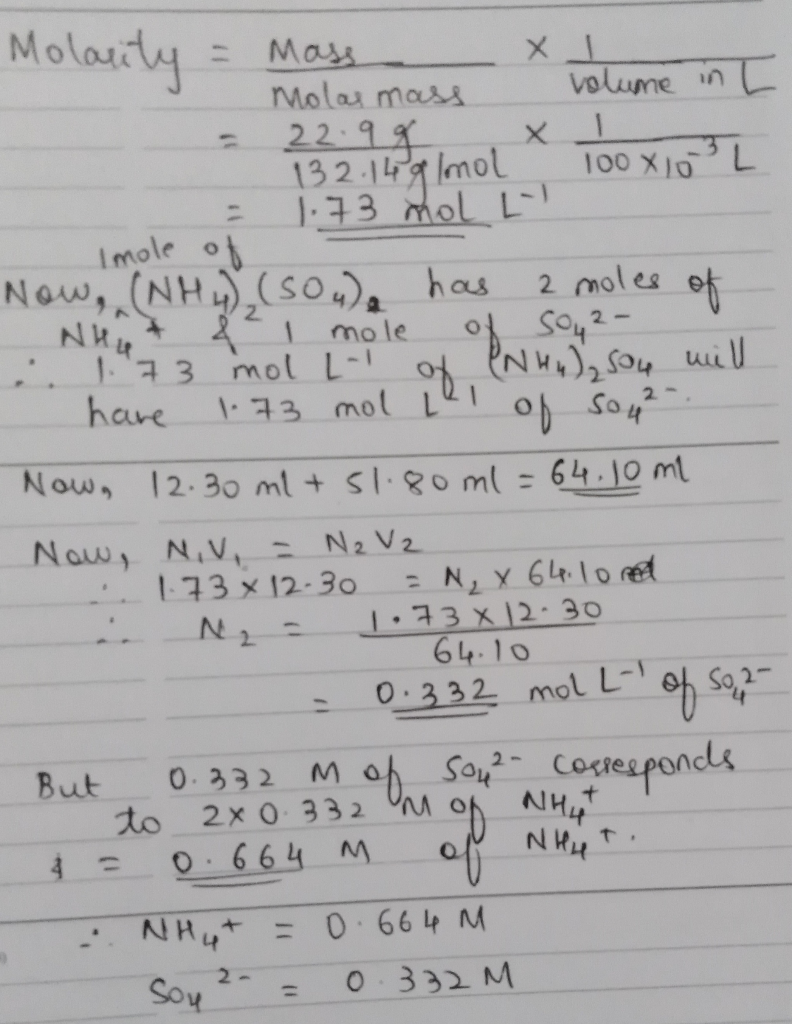Thank You!

#### Earn Coin

Coins can be redeemed for fabulous gifts.

Similar Homework Help Questions
• ### (References) A solution is prepared by dissolving 11.3 g ammonium sulfate in enough water to make...(References) A solution is prepared by dissolving 11.3 g ammonium sulfate in enough water to make 150.0 mL of stock solution. A 40.00-ml sample of this stock solution is added to 70.00 mL of water. Calculate the concentration of ammonium ions and sulfate ions in the final solution. Assume that the volumes are additive. M Concentration of ammonium ions - Concentration of sulfate ions - M Submit Answer Try Another Version 5 item attempts remaining ot

• ### 4) A buffer solution is prepared by dissolving 5.40 g of ammonium chloride in 20.0 mL...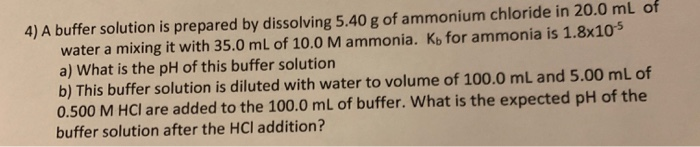4) A buffer solution is prepared by dissolving 5.40 g of ammonium chloride in 20.0 mL of water a mixing it with 35.0 mL of 10.0 Mammonia. Ky for ammonia is 1.8x10° a) What is the pH of this buffer solution b) This buffer solution is diluted with water to volume of 100.0 mL and 5.00 mL of 0.500 M HCl are added to the 100.0 mL of buffer. What is the expected pH of the buffer solution after the...

• ### When water is added to solids to prepare a solution, the volume of the solution is...

When water is added to solids to prepare a solution, the volume of the solution is not necessarily the same as the volume of the water added. For dilute solutions the two volumes are very similar. Assuming the volume of solutions prepared is 10.0 mL calculate the following based on the mass 0.2610 of ammonium sulfate. 1. The molarity of ammonium sulfate in the solution expressed to 3 decimal places. 2. the molarity of ammonium ions in the solution 3....

• ### Section: Name: Laboratory Questions: Solution Stoichiometry A solution is prepared by dissolving 3.7500 grams iron(III) oxalate...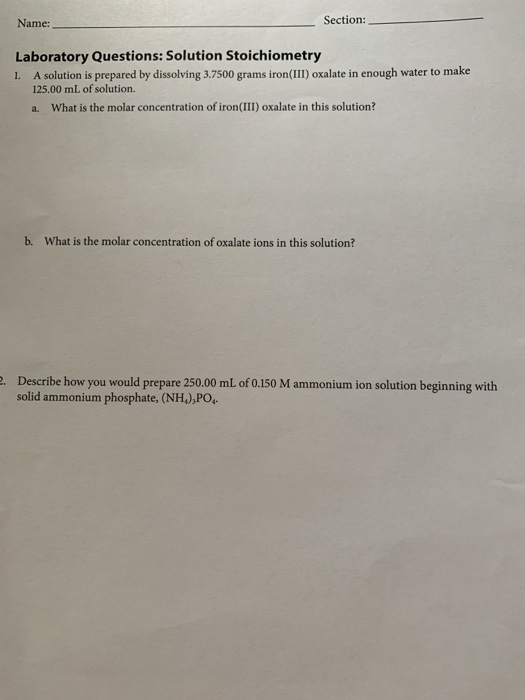Section: Name: Laboratory Questions: Solution Stoichiometry A solution is prepared by dissolving 3.7500 grams iron(III) oxalate in enough water to make 125.00 mL of solution. 1. What is the molar concentration of iron(III) oxalate in this solution? a. b. What is the molar concentration of oxalate ions in this solution? . Describe how prepare 250.00 mL of 0.150 M ammonium ion solution beginning with would you solid ammonium phosphate, (NH), PO

• ### A 40.0-ml solution contains 0.021 M barium chloride (BaCl2). What is the minimum concentration of sodium...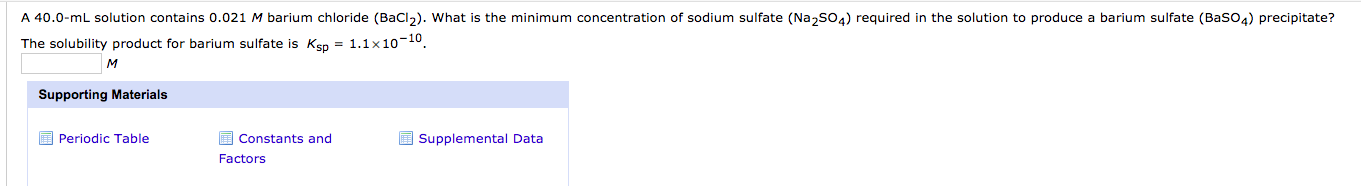A 40.0-ml solution contains 0.021 M barium chloride (BaCl2). What is the minimum concentration of sodium sulfate (Na2SO4) required in the solution to produce a barium sulfate (BaSO4) precipitate? The solubility product for barium sulfate is Ksp = 1.1x10-10 Supporting Materials Periodic Table Supplemental Data Constants and Factors

• ### Solution A is prepared by dissolving 89.4 g of Na3PO4 in enough water to make 1.50...

Solution A is prepared by dissolving 89.4 g of Na3PO4 in enough water to make 1.50 L of solution. Solution B is 2.5 L of 0.696 M Na2SO4. a) What is the molar concentration of Na3PO4 in Solution A?   0.36 M b) How many millilitres of Solution A will give 2.50 g of Na3PO4? 41.95 mL c) A 50.0 mL sample of Solution B is mixed with a 75.00 mL sample of Solution A. Calculate the concentration of Na+ ions...

• ### A student prepared a stock solution by dissolving 5.00 g of KOH in enough water to make 150. mL...

A student prepared a stock solution by dissolving 5.00 g of KOH in enough water to make 150. mL of solution. She then took 15.0 mL of the stock solution and diluted itwith enough water to make water to make 65.0 mL of a final solution. What is the concentration of KOH for the final solution?A) 0.137 MB) 7.30 MC)5.61 MD) 0.178 M

• ### A solution is prepared by dissolving 20.2 mL of methanol (CH3OH) in 100.0 mL of water...

A solution is prepared by dissolving 20.2 mL of methanol (CH3OH) in 100.0 mL of water at 25 ∘C. The final volume of the solution is 118 mL. The densities of methanol and water at this temperature are 0.782 g/mL and 1.00 g/mL, respectively. For this solution, calculate mole percent M=4.18 m=4.93 percent by mass= 13.6% mole fraction= 8.15*10^-2

• ### Calculate the concentrations of hydroxide and hydronium ions in a solution prepared by dissolving 0.471 g...

Calculate the concentrations of hydroxide and hydronium ions in a solution prepared by dissolving 0.471 g of solid NaOH in enough water to make 225 mL of solution. Kw = 1.0E-14. Hydroxide ion concentration? M The number of significant digits is set to 3; the tolerance is +/-4% LINK TO TEXT Hydronium ion concentration? M The number of significant digits is set to 3; the tolerance is +/-4%

• ### If 200. mL of water is added to 300. mL of an aqueous solution that is...

If 200. mL of water is added to 300. mL of an aqueous solution that is 0.64 M in potassium sulfate, what is the concentration of potassium ions in the final solution?